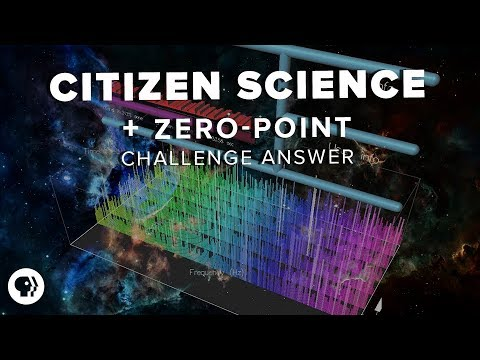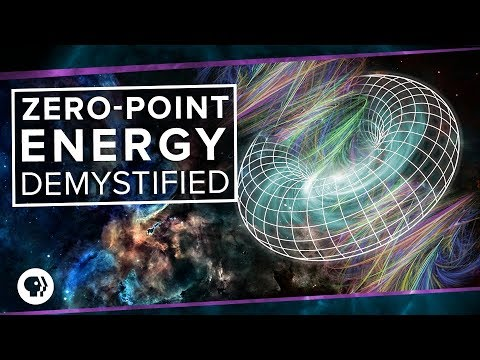# Search PBS Space Time

## Results### 2017-11-29: Citizen Science + Zero-Point Challenge Answer

• 07:18: In this theoretical estimate, vacuum energy density is proportional to the fourth power of cutoff frequency.
• 07:25: So my question was what cutoff frequency is needed for this theoretical estimate to give you the energy density implied by dark energy?
• 07:59: And if vacuum energy is proportional to the fourth power of cutoff frequency, then this equation gives the relationship we need.
• 08:07: There are a bunch of constants in the cutoff frequency vacuum energy relation, but they cancel out.
• 08:17: So we get a cutoff frequency for dark energy of 3 by 10 to the power of 12 hertz.### 2017-11-08: Zero-Point Energy Demystified

• 08:35: ... by integrating the energy in all possible frequency modes up to some cutoff frequency. ...
• 08:48: You get that the vacuum energy density is proportional to the fourth power of that cutoff frequency.
• 09:00: What cutoff frequency is needed for this theoretical estimate to give the energy density implied by dark energy.
2 result(s) shown.